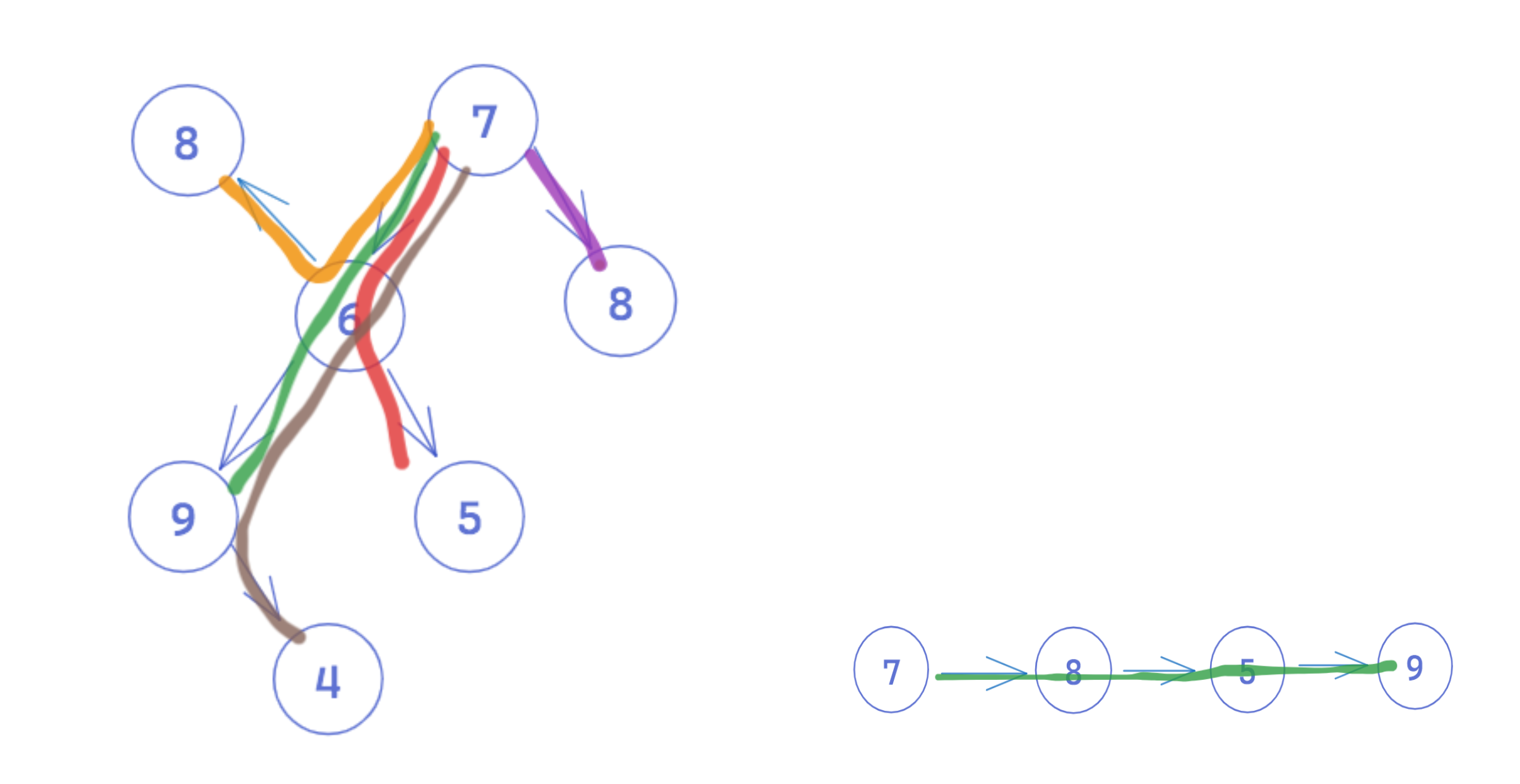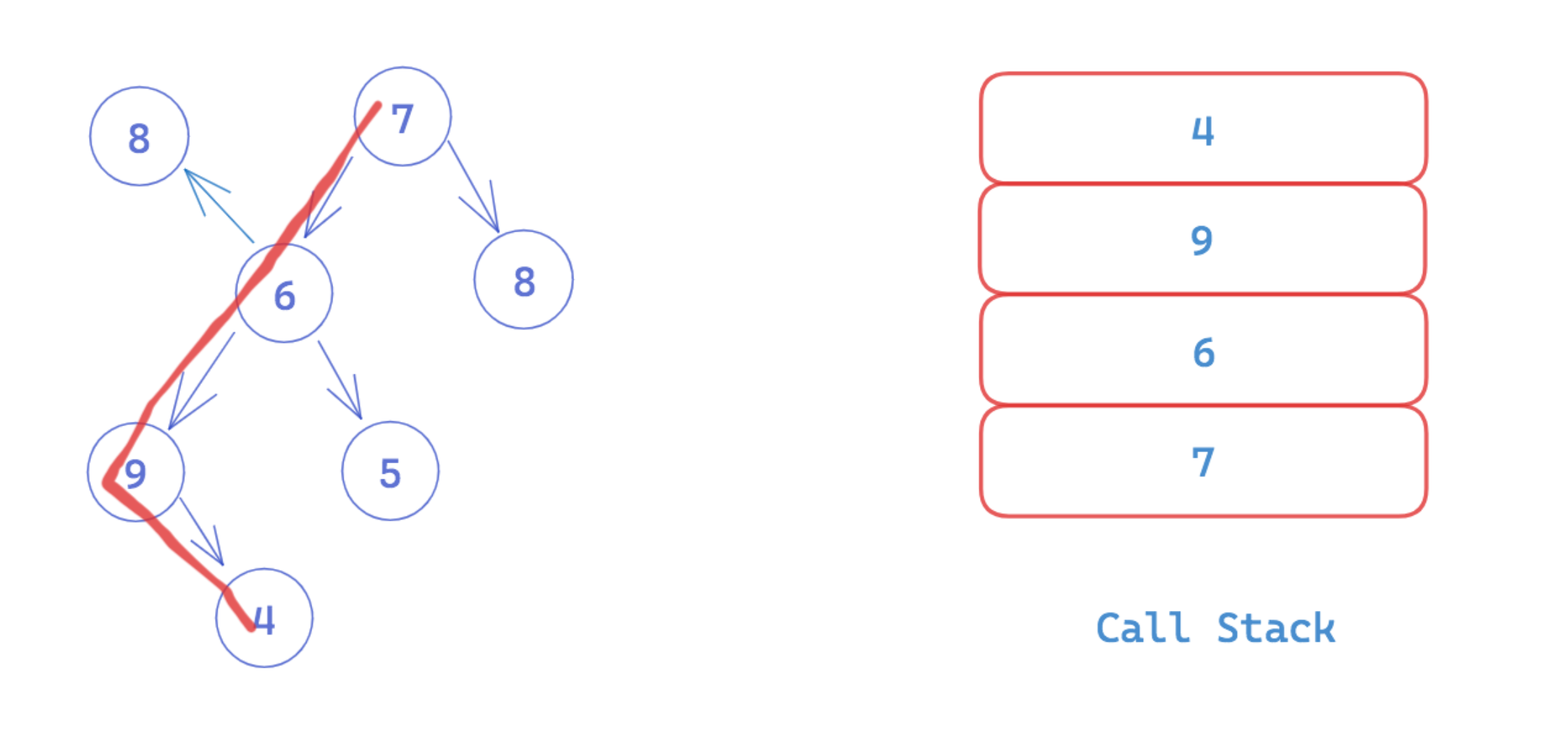# Depth-First Search (DFS)

By Kenny Polyak and The Mighty Anomaly | Published: June 30, 2023

An essential aspect of working with graphs and trees is understanding how to traverse the search space. Traversal is the process of systematically visiting each node exactly once following a specific order or pattern. This allows us to search for a node or to trace a specific path through the data structure.

Unlike a linear data structure like an array or a linked list - where each node points to only one subsequent node - graphs and trees offer multiple distinct paths to take through the structure. The example below illustrates the many paths that exist through a tree and the single path through a linked list.Different traversal algorithms will produce different traversal orders - knowing which to deploy and when enables us to solve problems more efficiently, and sometimes offers the only way to solve a particular problem. Let's look at how a specific traversal algorithm can be used! At the highest level, there are two main traversal algorithms: Depth-First Search (DFS), which is further distinguished with pre-order, in-order, and post-order traversal when specifically considering DFS in a binary tree, and Breadth-First Search (BFS). In this article, we'll focus on DFS.

Keep in mind that while we can manipulate the traversal path with different algorithms, unless the data structure is ordered in a particular way (like a BST) or our algorithm applies additional logic to omit certain paths, each traversal algorithm will ultimately visit each node once. DFS and BFS are considered blind search algorithms as they do not apply any domain-driven heuristic. Instead, the algorithms only apply traversal rules and a terminal case to determine if a goal state is reached.

Note: Since trees are merely directional, acyclic graphs, we'll just refer to both as graphs in the remainder of this article. Everything discussed below is relevant to trees as well as generic graphs, and in cases where that's not true, it will be pointed out as such.

## What is Depth-First Search (DFS)?

Depth-First Search (DFS) is a type of search algorithm that explores a graph by traversing as far as possible along each branch before backtracking. It's considered "depth-first" because at each node, if there are children, the algorithm always explores deeper paths until a leaf node is finally visited or some condition is met. The algorithm starts at the root node and explores the left and right subtrees by going deeper into the tree as it processes nodes, instead of visiting all the children of a given node before moving on.

There are many paths that DFS can take, given that each node can have multiple children. In fact, DFS is commonly used as a fundamental technique for backtracking algorithms - backtracking involves exploring all possible solutions to a problem (represented as paths through the data structure) by incrementally building a candidate path and undoing or "backtracking" when a solution is found to be invalid.

For binary trees, a very specific kind of graph, the order of the DFS has a big impact on the ultimate traversal order, and is very important when considering a DFS implementation. Learn more about pre-order, in-order, or post-order traversal in a binary tree here.

## DFS Implementation

### Recursive DFS

DFS is typically implemented with recursion, which works naturally with the recursive structure of a graph. We define a function that takes a node as an argument, which handles doing work on the current node (eg. printing the value) and initiating subsequent recursive calls on its children. Note that in some implementations, we will also use a set to track already visited nodes - this is important if we don't know if the graph is acyclic, otherwise we'll cause an infinite loop.

Here are the algorithm steps:

1. Create a `visited` set to keep track of visited nodes to avoid revisiting them.
2. Define a recursive function (`dfs`) that takes the current `Node` as a parameter.
3. Mark the current node as visited by adding it to the `visited` set.
4. Process the current node. This could involve performing any desired operations on the node or checking if it matches some search criteria.
5. Iterate through the `neighbors` of the current node. For each unvisited neighbor, recursively call the `dfs` function with that neighbor as the argument.
6. Repeat steps 3 to 5 for all unvisited neighbors until there are no more unvisited neighbors or the search criteria are met.

Imagine we have a graph with the following node structure:

``````class Node:
def __init__(self, id):
self._id = id
self._neighbors = []
``````

DFS will recursively call itself on each neighbor of the node. Below, we implement the algorithm using this adjacency list graph, but the same idea would apply for graphs represented using an adjacency matrix or a Von Neumann neighborhood. The neighbor retrieval would only be different.

Additionally, changing the order in which we iterate over the neighbors will also change the ultimate traversal order. This is especially important when dealing with binary search trees, where the nodes are sorted in some way.

``````class Graph:
def __init__(self):
self.visited = set()

def dfs(self, node):
# Mark the current node as visited

# Process the current node
print(node)

# Iterate through neighbors
for neighbor in node._neighbors:
if neighbor not in self.visited:
# Recursive call with unvisited neighbors
self.dfs(neighbor)

# Create a Graph object
g = Graph()
# Assuming that startNode is already defined
g.dfs(startNode)
``````

We begin the traversal by passing the root in as an argument. The function calls itself by passing in the current node's neighbors as arguments for subsequent calls, and a new instance of the function is instantiated as a new frame on the call stack.By using a recursive function, the algorithm keeps track of the nodes that need to be visited with the use of the call stack. When each call eventually returns – meaning, all child nodes have been traversed – the algorithm is effectively transported back to the moment that a given call was initialized.

### Iterative DFS

Although the structure of graphs lend themselves to recursive algorithms, this does not mean that one cannot also use iteration to traverse. Recursion is used in order to take advantage of the call stack - but we can also implement a stack of our own, removing the need for recursion.

Iterative approaches to DFS traversal involve using a loop and a stack for traversal. Using a while loop to iterate, a stack can be used to keep track of the nodes that still need to be visited, ensuring that the most recently added nodes, the closest parents, are visited first. This approach allows for efficient traversal of the tree and requires slightly less memory than recursive approaches, albeit no asymptotic change occurs.

``````class Graph:
def __init__(self):
self.visited = set()

def dfs(self, node):
stack = [node]

while stack:
# Pop the top node from the stack
current_node = stack.pop()

if current_node not in self.visited:
# Mark the current node as visited

# Process the current node
print(current_node)

for neighbor in current_node._neighbors:
# Push unvisited neighbors onto the stack
stack.append(neighbor)

# Create a Graph object
g = Graph()
# Assuming that startNode is already defined
g.dfs(startNode)
``````

#### Time and Space Complexity

Time complexity: `O(V + E)`, where `V` is the number of vertices and `E` is the number of edges, as it must process each vertex and each edge exactly once. Space complexity: `O(V`), where `V` is the number of vertices, as it requires a position in the stack for each vertex.

## When to Use DFS in Technical Interviews

Recall that in an acyclic graph, or a tree, all traversal algorithms will visit each node at least once, so for many problems that require basic search, both DFS and BFS will suffice. But there are certain problems where DFS is preferable:

• DFS is particularly useful for backtracking algorithms: When we need to explore all possible paths, and reverse course when a leaf node or some other condition is met, DFS allows for a convenient way to analyze various paths. Examples of problems like this are those where we are calculating the path sum - adding together the values of the nodes - either searching for the max or optimal sum.
• When there could be an opportunity to cache duplicate operations: If you're dealing with a graph or a tree that might include overlapping subproblems, you'll want to implement your traversal with DFS so it's easy to cache or memoize the sub-solutions. A classic example of a memoization use-case is when solving fibonacci with recursion - this algorithm effectively imagines the fibonacci resolution as a decision tree which is traversed with a DFS-like recursive algorithm.
• If there is an exponential branching factor: Memoized DFS is also important to consider if the branching factor of the recursion is exponential, as the volume of computations could be costly.
• In most cases, DFS is slightly more memory efficient than BFS, (though not always): This is worth mentioning if you're in a situation where both algorithms are applicable. In a tree, the space usage for DFS is defined as `O(height)`, where height is effectively the number of levels in the tree. BFS, on the other hand, uses `O(branching factor ^ height)`, where `branching factor` is the max or average number of children per node. As such, if `height < branchingFactor ^ height`, then DFS is more space efficient. This is rarely not the case. The absolute time complexity is unlikely to change here though, so the difference in an asymptotic sense is negligible.

## Common Mistakes in Interviews Featuring DFS

• Misunderstanding the tradeoffs and benefits between DFS and BFS: Remember that in many cases both will do just fine, with little to no tradeoffs, but there are specific cases when we should use BFS (finding the shortest distance between two nodes, for example).
• Not using the correct supporting data structure: BFS is made possible with a queue data structure, while DFS relies on a stack. It's important to be able to identify this when implementing, and to be sure not to use the wrong supporting data structure.
• Forgetting to track visited nodes: When dealing with a graph that is not guaranteed to be directed and acyclic, it is essential to track visited nodes in order to avoid an infinite loop. Interviewers will be looking for this as a common pitfall. One way to ensure you don't forget is to perform a manual dry-run of your algorithm.
• Choosing BFS over DFS when it's not needed: Although in many cases these are both interchangeable, one big benefit of using DFS for backtracking algorithms is that memoization can be implemented easily.

## Adjacent Topics to Depth-First Search (DFS)Kenny Polyak

Kenny is a software engineer and technical leader with four years of professional experience spanning Amazon, Wayfair, and U.S. Digital Response. He has taught courses on Data Structures and Algorithms at Galvanize, helping over 30 students land new software engineering roles across the industry, and has personally received offers from Google, Square, and TikTok.The Mighty Anomaly

The Mighty Anomaly (Mike) is one of the top Google coaches for interviewing.io having personally helped over 100+ engineers get into Google with his unique coaching style. After receiving offers from the full FAANG gauntlet early in his career, he enjoys teaching others proven techniques to pass technical interviews with his decade of coaching and industry experience.